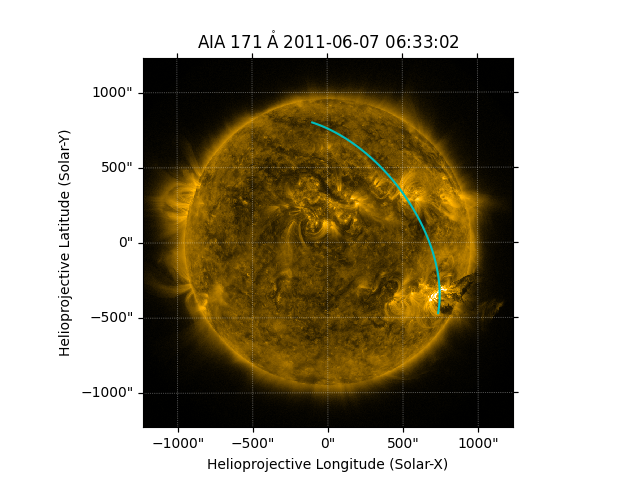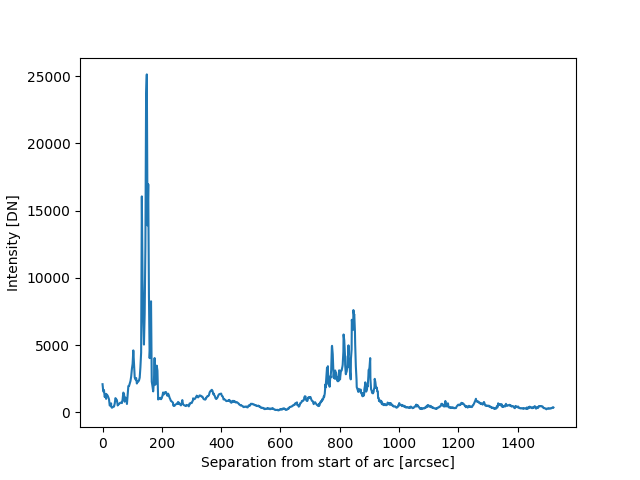# Drawing and using a Great Arc¶

How to define and draw a great arc on an image of the Sun, and to extract intensity values along that arc.

```import numpy as np
import matplotlib.pyplot as plt

import astropy.units as u
from astropy.coordinates import SkyCoord

import sunpy.map
from sunpy.coordinates.utils import GreatArc
from sunpy.data.sample import AIA_171_IMAGE
```

We start with the sample data

```m = sunpy.map.Map(AIA_171_IMAGE)
```

Let’s define the start and end coordinates of the arc.

```start = SkyCoord(735 * u.arcsec, -471 * u.arcsec, frame=m.coordinate_frame)
end = SkyCoord(-100 * u.arcsec, 800 * u.arcsec, frame=m.coordinate_frame)
```

Create the great arc between the start and end points.

```great_arc = GreatArc(start, end)
```

Plot the great arc on the Sun.

```fig = plt.figure()
ax = plt.subplot(projection=m)
m.plot(axes=ax)
ax.plot_coord(great_arc.coordinates(), color='c')
plt.show()
```Now we can calculate the nearest integer pixels of the data that correspond to the location of arc.

```pixels = np.asarray(np.rint(m.world_to_pixel(great_arc.coordinates())), dtype=int)
x = pixels[0, :]
y = pixels[1, :]
```

Get the intensity along the arc from the start to the end point.

```intensity_along_arc = m.data[y, x]
```

Define the angular location of each pixel along the arc from the start point to the end.

```angles = great_arc.inner_angles().to(u.deg)
```

Plot the intensity along the arc from the start to the end point.

```fig, ax = plt.subplots()
ax.plot(angles, intensity_along_arc)
ax.set_xlabel('degrees of arc from start')
ax.set_ylabel('intensity')
ax.grid(linestyle='dotted')
plt.show()
```Total running time of the script: ( 0 minutes 0.741 seconds)

Gallery generated by Sphinx-Gallery